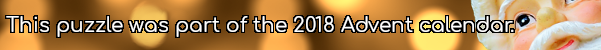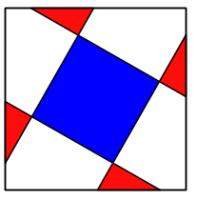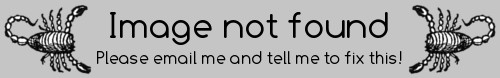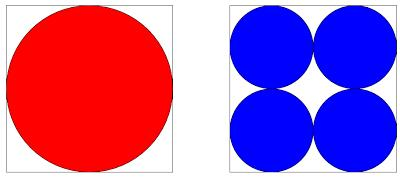mscroggs.co.uk
mscroggs.co.uksubscribe

# Puzzles

## 23 DecemberToday's number is the area of the largest area rectangle with perimeter 46 and whose sides are all integer length.

## 2 DecemberToday's number is the area of the largest dodecagon that it's possible to fit inside a circle with area $$\displaystyle\frac{172\pi}3$$.

## Squared circle

Each side of a square has a circle drawn on it as diameter. The square is also inscribed in a fifth circle as shown.
Find the ratio of the total area of the shaded crescents to the area of the square.

## Two triangles

Source: Maths Jam
The three sides of this triangle have been split into three equal parts and three lines have been added.
What is the area of the smaller blue triangle as a fraction of the area of the original large triangle?

## Overlapping triangles

Four congruent triangles are drawn in a square.
The total area which the triangles overlap (red) is equal to the area they don't cover (blue). What proportion of the area of the large square does each (purple) triangle take up?Let $$S$$ be the area of the large square, $$T$$ be the area of one of the large triangles, $$U$$ be one of the red overlaps and V be the uncovered blue square. We can write $$S=4T-4U+V$$ as the area of the square is the total of the four triangles, take away the overlaps as they have been double counted, add the blue square as it has been missed.
We know that 4U=V, so
$$S=4T-V+V$$ $$S=4T.$$
Therefore one of the triangles covers one quarter of the square.

#### Extension

Five congruent triangles are drawn in a regular pentagon. The total area which the triangles overlap (red) is equal to the area they don't cover (blue). What proportion of the area of the large pentagon does each triangle take up?$$n$$ congruent triangles are drawn in a regular $$n$$ sided polygon. The total area which the triangles overlap is equal to the area they don't cover. What proportion of the area of the large $$n$$ sided polygon does each triangle take up?

## Unit octagon

The diagram shows a regular octagon with sides of length 1. The octagon is divided into regions by four diagonals. What is the difference between the area of the hatched region and the area of the region shaded grey?

## Largest triangle

What is the largest area triangle which has one side of length 4cm and one of length 5cm?

## Circles

Which is largest, the red or the blue area?Let $$4x$$ be the side length of the square. This means that the radius of the red circle is $$2x$$ and the radius of a blue circle is $$x$$. Therefore the area of the red circle is $$4\pi x^2$$.
The area of one of the blue squares is $$\pi x^2$$ so the blue area is $$4\pi x^2$$. Therefore the two areas are the same.

#### Extension

Is the red or blue area larger?## Archive

Show me a random puzzle
▼ show ▼